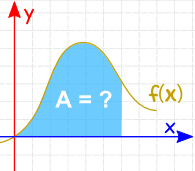# Integration Rules

## IntegrationIntegration can be used to find areas, volumes, central points and many useful things. It is often used to find the area underneath the graph of a function and the x-axis.

The first rule to know is that integrals and derivatives are opposites!Sometimes we can work out an integral,
because we know a matching derivative.

### Integration Rules

Here are the most useful rules, with examples below:

Common Functions Function Integral
Constant a dx ax + C
Variable x dx x2/2 + C
Square x2 dx x3/3 + C
Reciprocal (1/x) dx ln|x| + C
Exponential ex dx ex + C
ax dx ax/ln(a) + C
ln(x) dx x ln(x) − x + C
Trigonometry (x in radians) cos(x) dx sin(x) + C
sin(x) dx -cos(x) + C
sec2(x) dx tan(x) + C

Rules Function
Integral
Multiplication by constant cf(x) dx cf(x) dx
Power Rule (n≠−1) xn dx xn+1n+1 + C
Sum Rule (f + g) dx f dx + g dx
Difference Rule (f - g) dx f dx - g dx
Integration by Parts See Integration by Parts
Substitution Rule See Integration by Substitution

## Examples

### Example: what is the integral of sin(x) ?

From the table above it is listed as being −cos(x) + C

It is written as:

sin(x) dx = −cos(x) + C

### Example: what is the integral of 1/x ?

From the table above it is listed as being ln|x| + C

It is written as:

(1/x) dx = ln|x| + C

The vertical bars || either side of x mean absolute value, because we don't want to give negative values to the natural logarithm function ln.

### Example: What is ∫x3 dx ?

The question is asking "what is the integral of x3 ?"

We can use the Power Rule, where n=3:

xn dx = xn+1n+1 + C

x3 dx = x44 + C

### Example: What is ∫√x dx ?

√x is also x0.5

We can use the Power Rule, where n=0.5:

xn dx = xn+1n+1 + C

x0.5 dx = x1.51.5 + C

### Example: What is ∫6x2 dx ?

We can move the 6 outside the integral:

6x2 dx = 6x2 dx

And now use the Power Rule on x2:

= 6 x33 + C

Simplify:

= 2x3 + C

### Example: What is ∫(cos x + x) dx ?

Use the Sum Rule:

(cos x + x) dx = cos x dx + x dx

Work out the integral of each (using table above):

= sin x + x2/2 + C

### Example: What is ∫(ew − 3) dw ?

Use the Difference Rule:

(ew − 3) dw =ew dw − 3 dw

Then work out the integral of each (using table above):

= ew − 3w + C

### Example: What is ∫(8z + 4z3 − 6z2) dz ?

Use the Sum and Difference Rule:

(8z + 4z3 − 6z2) dz =8z dz + 4z3 dz − 6z2 dz

Constant Multiplication:

= 8z dz + 4z3 dz − 6z2 dz

Power Rule:

= 8z2/2 + 4z4/4 − 6z3/3 + C

Simplify:

= 4z2 + z4 − 2z3 + C